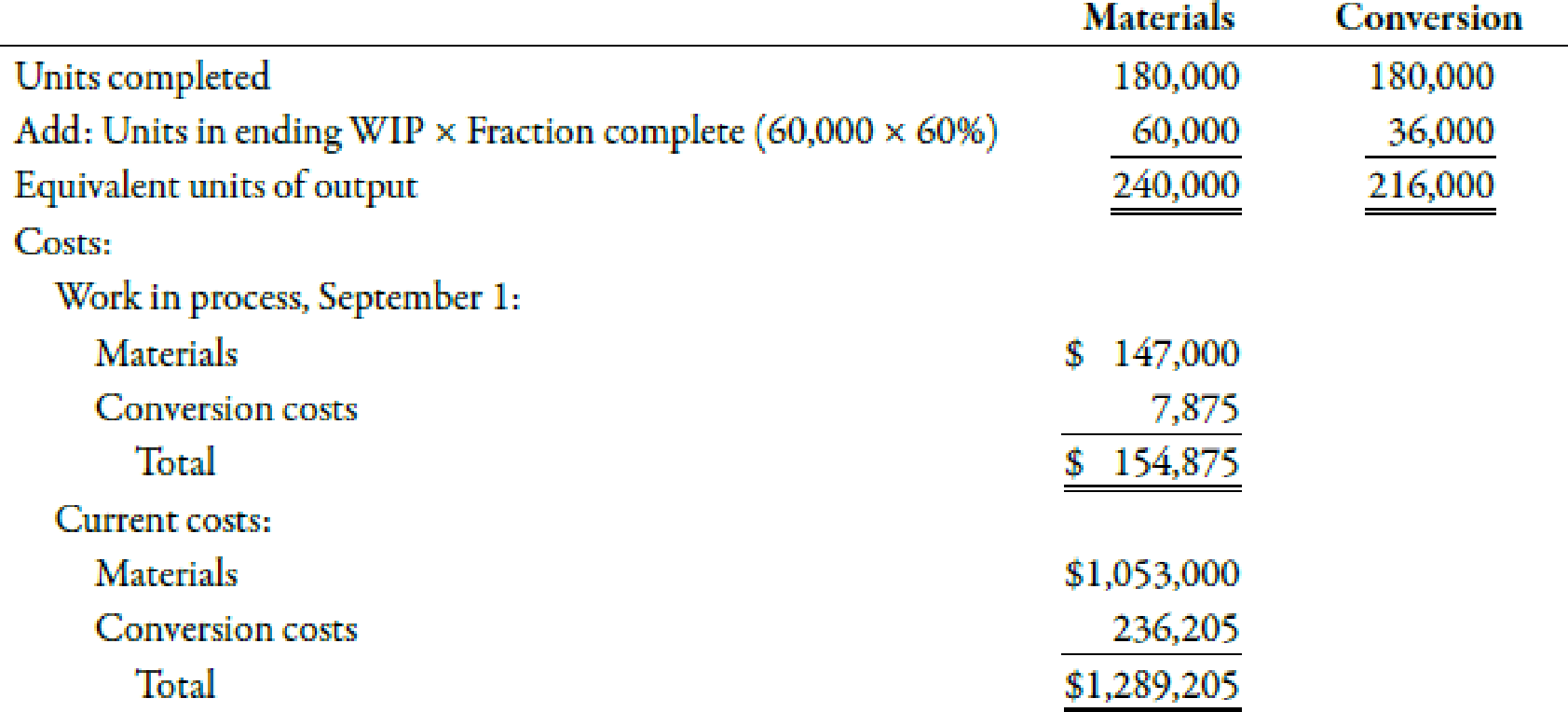Chapter 6, Problem 51E### Managerial Accounting: The Corners...

7th Edition
Maryanne M. Mowen + 2 others
ISBN: 9781337115773

#### Solutions

Chapter
Section### Managerial Accounting: The Corners...

7th Edition
Maryanne M. Mowen + 2 others
ISBN: 9781337115773
Textbook Problem
1 views

# Unit Cost and Cost Assignment, Nonuniform InputsLoran Inc. had the following equivalent units schedule and cost for its fabrication department during September:Required: 1. Calculate the unit cost for materials, for conversion, and in total for the fabrication department for September. 2. Calculate the cost of units transferred out and the cost of EWIP.

1.

To determine

Compute the unit cost for materials, conversion and total unit cost.

Explanation

Equivalent Units of Production:

The total units of finished goods along with those goods that are not fully complete are known as equivalent units of production. In other words, equivalent units of production measures both completed and nearly completed units.

Use the following formula to calculate unit material cost:

Unit material cost=(Cost of BWIP for material+Current material costEquivalent units)=($147,000+$1,053,000240,000 units)=$5.00 Therefore, the unit material cost is$5.00

2.

To determine

Compute the cost of goods transferred out and cost of EWIP of Company LI

### Still sussing out bartleby?

Check out a sample textbook solution.

See a sample solution

#### The Solution to Your Study Problems

Bartleby provides explanations to thousands of textbook problems written by our experts, many with advanced degrees!

Get Started

#### Find more solutions based on key concepts